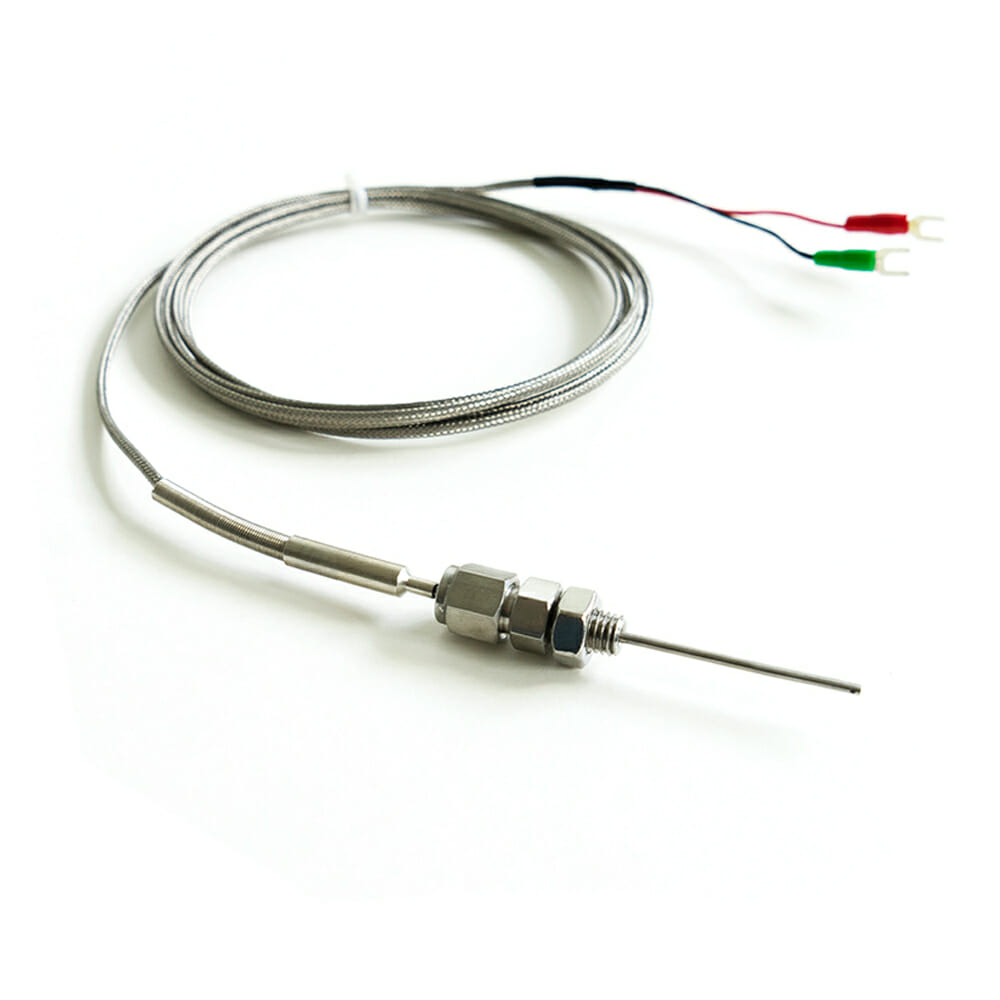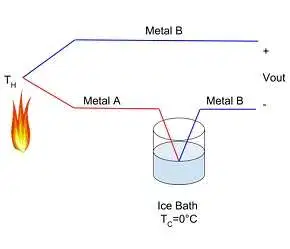## Thermocouples

How does a thermocouple work?Hint
A thermocouple is a sensor that measures temperature.
The fundamental idea of a thermocouple is that two dissimilar metals are joined together at their two ends. If one end is placed on something hot, and the other end is placed on something cold, a potential difference, or voltage, develops. This difference can be measured by placing a voltmeter across the two hot/cold junctions.The phenomenon of current flowing though two different temperature metals is known as the Seebeck effect, or thermoelectric effect.

In the specific case of the thermocouple, we can first calibrate the instrument by measuring known temperatures to find the mathematical formula, or relationship, between the current and temperature. Once calibrated, the device can then measure unknown temperatures by placing one metal end at the unknown, and the other metal junction on an object that is in a precisely known temperature range. The outputted voltage difference can be plugged into the pre-determined formula to calculate the desired variable.

The fundamental idea of a thermocouple is that two dissimilar metals are joined together at their two ends. If one end is placed on something hot, and the other end is placed on something cold, a potential difference, or voltage, develops. This difference can be measured by placing a voltmeter across the two hot/cold junctions.